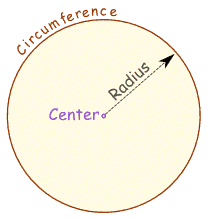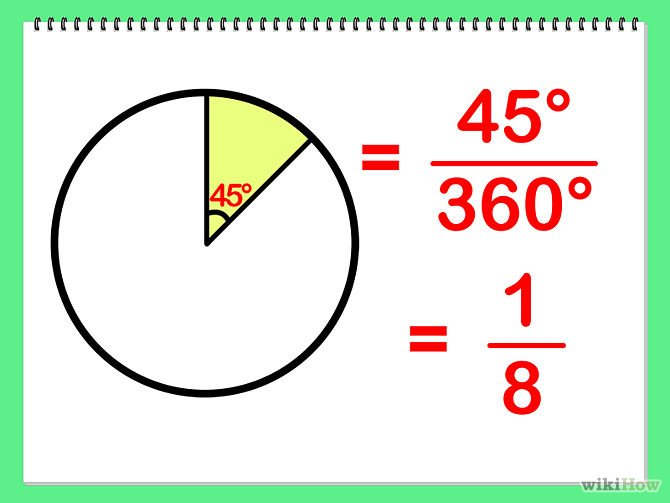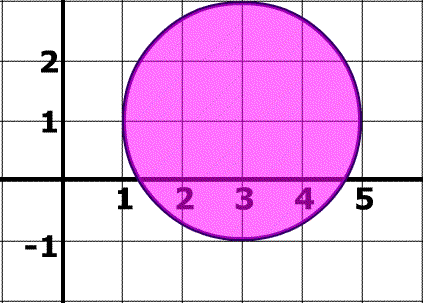Give us feedbackX

# Circle Formulas and Equations

09 Sep 2015Homework Help Online

Have you ever seen a circle? This two-dimensional shape is in a clock, base of a glass, and a coin. A circle is a set of points on a particular plane with same, fixed distance from its center. In mathematics, there are some familiar circle terms, such as radius (radii), diameter, and circumference. You probably will need math help about circle formulas and equations for your homework. Don’t worry, you can learn more and understand about circle formulas and equations in the following passages.

### What are radius, diameter, and circumference?Credit: math is fun

Radius or radii (r) is the distance from the center to a point of the circle. Radius always the same value for all points. Diameter (d) is the distance from a point to another point through the center. Its value is twice of radius. Circumference is the distance around your circle.

How do we calculate area and circumference of a circle?

A = πr2 = π ( d/2 )2

C = πd = 2πr

The symbol π (pi) is a Greek letter with value of 3.14 (rounded). It is a magical number which has to be remembered every time you deal with circle.

### Example:

r = 20 cm

A = πr2 = π x 202 = 3.14 x 400 = 1,256 cm2

C = 2πr = 2 x 3.14 x 20 = 125.6 cm

## How do we calculate the sector of a circle?

Imagine a sector as a slice of pizza, a part of a big circle.Credit: wikiHow

A = πr2 ( n/360 )

With n is the sector angle.

## How do we calculate circle equations?

There are two forms of circle equations:

### 1. Standard formCredit: math is fun

(x – a)2 + (y – b)2 = r2

(x, y) is your point and (a, b) is the center.

### 2. General form

General form is an extended version of standard form.

x2 + y2 + Ax + By + C = 0

A, B, and C are constants.

Examples:

Find the circle equation!

1.Credit: math warehouse

(a, b) = (1, 0)

r = 1

Solution:

(x – a)2 + (y – b)2 = r2

(x – 1)2 + (y – 0)2 = 12 (standard form)

(x2 – 2x + 1) + y2 = 1

x2 + y2 – 2x = 0 (general form)

2.Credit: math warehouse

(a, b) = (3, 1)

r = 2

Solution:

(x – a)2 + (y – b)2 = r2

(x – 3)2 + (y – 1)2 = 22

(x – 3)2 + (y – 1)2 = 4 (standard form)

(x2 – 6x + 9) + (y2 – 2y + 1) = 4

x2 + y2 – 6x – 2y + 9 + 1 = 4

x2 + y2 – 6x – 2y + 6 = 0 (general form)

You can use these circle formulas and equations when do your homework, calculate area or circumference of a circular land or fence, even make a craftwork! They can be applied to almost all things related to circle or circular objects.

## Other recommended websites to read:

You can read about standard and general forms of circle equation with simple illustrations. This web also shows you how to plot a circle.

To know how to calculate the area of circle using various ways, you may find this site useful. Real examples are also given using real objects.

This webpage contains various methods to find circumference. Accompanied with clear illustrations, it will guide you step by step.

Here, you’ll learn about the definition of a circle and the circle equation. Examples of the problems are given along with the solutions.

This math web brings you the definition of circle, radius, chord, diameter, arc, and semicircle. In-depth learning about angle is also available.

Related Posts

Testimonials

• Jason L.

I have a lot of questions that need to be answered. While searching online, I found the site of StudyFAQ and it is all worth it. The tutor is very kind and I’ve got everything I needed. Thank you so much.

• Garry T.

Tutor did a great job. The process was very fast and efficient. I didn’t know that there is a quality and reliable tutoring service provider online. I can now start with my assignment.

• Margret O.

I was really having a hard time finding an expert who can help me with my topic. Thanks to StudyFAQ.com, I can start working on my assignment now.